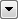You are here: ManipulatorsAffiner

# Affiner

Performs an affine transformation on the coordinates of the feature.

An affine transformation preserves lines and parallelism in geometry. That is, any lines that were parallel before the transformation are parallel after the transformation. In addition, if a number of points falling on a straight line are transformed, the resulting coordinates will fall on a straight line in the new coordinate system.

Affine transformations include translations, rotations, scalings, and reflections.

## Examples

### Translating Features East and North

To move features 500 units east and 1000 units north, use the following formula:

x' = 1x + 0y + Easting

y' = 0x + 1y + Northing

x' = 1x + 0y + 500

x' = 1x + 0y + 500

You would then enter the corresponding values for A to F in the Affiner parameters (for example, A=1, B=0, C=500, D=0, E=1, F=1000)

### Scaling a Feature Set

To scale features by factor R, use the scale factor to the X and Y terms as follows:

x' = Rx + 0y + 0

y' = 0x + Ry + 0

To increase the scale by 10 times:

x' = 10x + 0y + 0

y' = 0x + 10y + 0

### Rotating Features

To rotate features, apply the appropriate cos and sin functions associated with the rotation angle to the X,Y coefficients:

x' = cos(w)x + -sin(w)y + 0

y' = sin(w)x + cos(w)y + 0

where w = angle of rotation.

So, for a rotation of 30 degrees, cos(30) = 0.866025, sin(30) = 0.5, you would use:

x' = 0.866025*x + -0.5*y + 0

y' = 0.5*x + 0.866025*y + 0

to rotate a set of features by 30 degrees.

### Combining Transformations

You can combine a set of transformations into one formula, or perform them in series to make it easier to debug or edit. For example, it may be easier to do your rotation and then scaling in two different Affiner transformers rather than trying to combine them into one transformation. Naturally, one advantage to doing it all in one transformation would be speed.

Note: Hint: Right-click on the transformer in the workspace and click Show Summary Annotation. This makes it easy to preview the transformation formula that will be used.

## Usage Notes

• Use the Affiner, rather than the Scaler, when scaling rasters. Because raster data is scaled around the data origin, not the coordinate origin, using the Scaler can cause an unexpected data shift).

## Related Transformers

• Please also see the AffineWarper, which performs warping operations on the spatial coordinates of features. The AffineWarper is used to adjust a set of observed features so they more closely match some set of reference features.
• The Scaler and Offsetter are both simplified versions of the Affiner. For example:

x' = Ax + By + C

and

y' = Dx + Ey + F

where A = 1000 and E = 1000

In this case, x' = 1000x and y' = 1000y is the same as Scaler.

## Editing Transformer Parameters

Using a set of menu options, transformer parameters can be assigned by referencing other elements in the workspace. More advanced functions, such as an advanced editor and an arithmetic editor, are also available in some transformers. To access a menu of these options, clickbeside the applicable parameter. For more information, see Transformer Parameter Menu Options.

## Search FME Knowledge Center

Search for samples and information about this transformer on the FME Knowledge Center.

Tags Keywords: pointcloud